[BACK]
 Journal of New MediaDOI:10.32604/jnm.2022.027060Article

Research on Image Quality Enhancement Algorithm Using Hessian Matrix

1China Mobile Group Henan Co., Ltd., Zhengzhou, 450000, Henan, China
2Department of Information Science and Engineering, Hunan First Normal University, Changsha, 410205, Hunan, China
3Department of Information Engineering, Shaoyang University, Shaoyang, 422000, Hunan, China
*Corresponding Author: Yanpeng Wu. Email: xjzxwyp@hnfnu.edu.cn
Received: 09 January 2022; Accepted: 15 April 2022

Abstract: The Hessian matrix has a wide range of applications in image processing, such as edge detection, feature point detection, etc. This paper proposes an image enhancement algorithm based on the Hessian matrix. First, the Hessian matrix is obtained by convolving the derivative of the Gaussian function. Then use the Hessian matrix to enhance the linear structure in the image. Experimental results show that the method proposed in this paper has strong robustness and accuracy.

Keywords: Hessian matrix; Gaussian function; image enhancement; linear structure

1  Introduction

Hessian matrix has a wide range of applications in image processing , such as edge detection  and feature point detection . The Hessian matrix itself also contains a lot of mathematical knowledge , such as Taylor expansion, multivariate function derivation, matrix, eigenvalues, etc.

2  Taylor Expansion and Hessian Matrix

Performing Taylor expansion on the unary function f(x) at x0, the following formula can be obtained.

f(x0+δx)=f(x0)+δxf(x0)+12δx2f(x0)+oδ x2 (1)

Among them, the remainder is the Peano remainder, and the part of the second derivative is mapped to the two-dimensional and multi-dimensional space as the Hessian matrix.

In a two-dimensional image, assuming that the function of the image pixel value relative to the coordinates (x, y) is f(x, y), then expand f(x + dx, y + dy)) at f(x0, y0) to get as follows:

f(x0+δx,y0+δy)=f(x0,y0)+δxfx(x0,y0)+δyfy(x0,y0)+12δx2fxx(x0,y0)       +2δxδyfxy(x0,y0)+δy2fyy(x0,y0)+o(2)

Eq. (2) is expressed by a matrix, and the remainder is discarded, then:

f(x,y)f(x0,y0)+[δxδy][fx(x0,y0)fy(x0,y0)]+12[δxδy][fxx(x0,y0)fxy(x0,y0) fyx(x0,y0)fyy(x0,y0)][δxδy](3)

The second matrix in the third term on the right side of the above formula is the Hessian matrix in two-dimensional space; therefore, we conclude that the Hessian matrix is the second derivative of a certain point in the space. To summarize further, the Hessian matrix in the multidimensional space can be expressed as:

H=[2fx1x12fx1x22fx2x12fx1xn2fx2xn2fxnx12fxnxn](4)

As we all know, the second derivative represents the change law of the derivative. If the function is a curve and the curve has a second derivative, then the second derivative represents the curvature of the curve. The greater the curvature, the more curved the curve. By analogy, the second derivative of a point in the multidimensional space represents the speed at which the gradient drops at that point. Taking a two-dimensional image as an example, the first-order derivative is the grayscale change of the image, that is, the grayscale gradient, and the second-order derivative is the degree of grayscale gradient change. The larger the second derivative, the larger the gray gradient change.

But in a two-dimensional image, the Hessian matrix is a two-dimensional positive definite matrix with two eigenvalues and two corresponding eigenvectors. These two eigenvalues represent the anisotropy of the image in the direction pointed by the two eigenvectors. If an ellipse is composed of eigenvectors and eigenvalues, then this ellipse marks the anisotropy of the image change. So in a two-dimensional image, what kind of structure is the most isotropic and what kind of structure is more anisotropic? Obviously, the isotropy of the circle is the strongest, and the structure with the stronger linearity is more anisotropic.

The characteristics that the eigenvalues should have are shown in Tab. 1.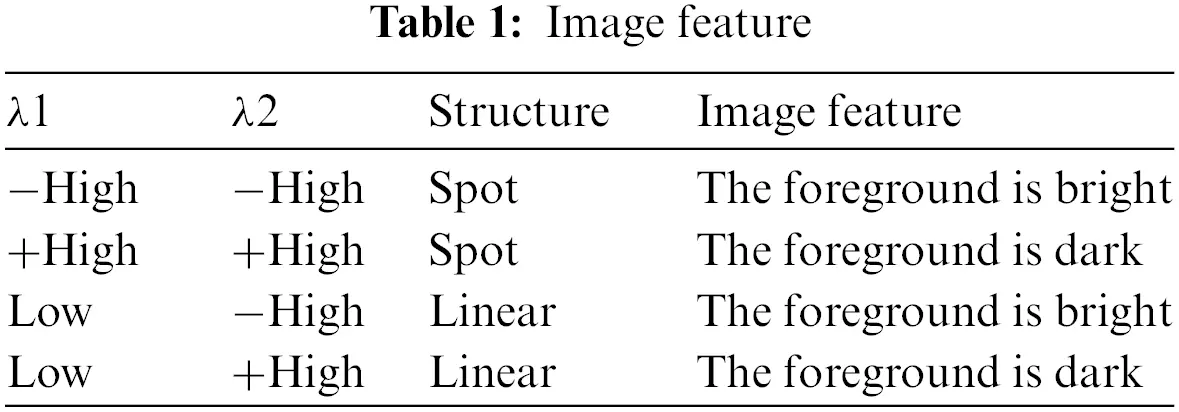3  Application of Hessian Matrix

The Hessian matrix has many applications. The ellipse formed by the eigenvalues of the matrix and the eigenvector represents the anisotropy of the image, and this anisotropic image processing is applied. Taking a two-dimensional image as an example, the point structure in the image is isotropic, while the linear structure is anisotropic. Therefore, the Hessian matrix can be used to enhance the linear structure in the image and filter out point-like structures and noise points. Similarly, it can also be used to find the point structure in the image and filter out other information.

Of course, when using the Hessian matrix, there is no need to perform Taylor expansion on the image, only the elements in the matrix need to be directly obtained. Generally, the second derivative of a digital image uses the following formula:

fxx(x,y)=f(x,y)f(x+δx,y)(f(x+δx,y)f(x+2δx,y))(5)

However, this method has poor robustness and is susceptible to interference from local signals in the image. It can even be said that this derivation method is controversial. You can also use the following formula to calculate the second derivative:

fxx(x,y)=f(x,y)f(xδx,y)(f(xδx,y)f(x2δx,y))(6)

In addition to the above two expression methods, the second derivative can also be expressed in other ways. Different methods often get different values, because this method only contains the information of three points including itself. not enough. According to the linear scale space theory (LOG), the derivative of a function is equal to the convolution of the derivative of the function and the Gaussian function. The formula is as follows:

f(x,y)x=f(x,y)G(x,y)x(7)

Since the Gaussian template can contain the information of all points in the surrounding rectangle, there will be no errors. Therefore, when using the image to obtain the elements in the hessian matrix, the image can be convolved with the second derivative of the Gaussian function. The second-order partial derivative formula of Gaussian function is as follows:

2G(x,y)x2=12πσ4(1x2σ2)ex2+y22σ2(8)

2G(x,y)y2=12πσ4(1y2σ2)ex2+y22σ2(9)

2G(x,y)xy=xy2πσ6ex2+y22σ2(10)

When writing a program, you need to convolve the image on three templates in advance to generate a “graph” of three partial derivatives, and then index the partial derivatives of the corresponding positions each time as needed.

4  Experimental Analysis

Use the algorithm in this paper to carry out an image quality enhancement experiment on a frame of ordinary pictures. Fig. 1 is the original image, and Figs. 25 is the enhanced image.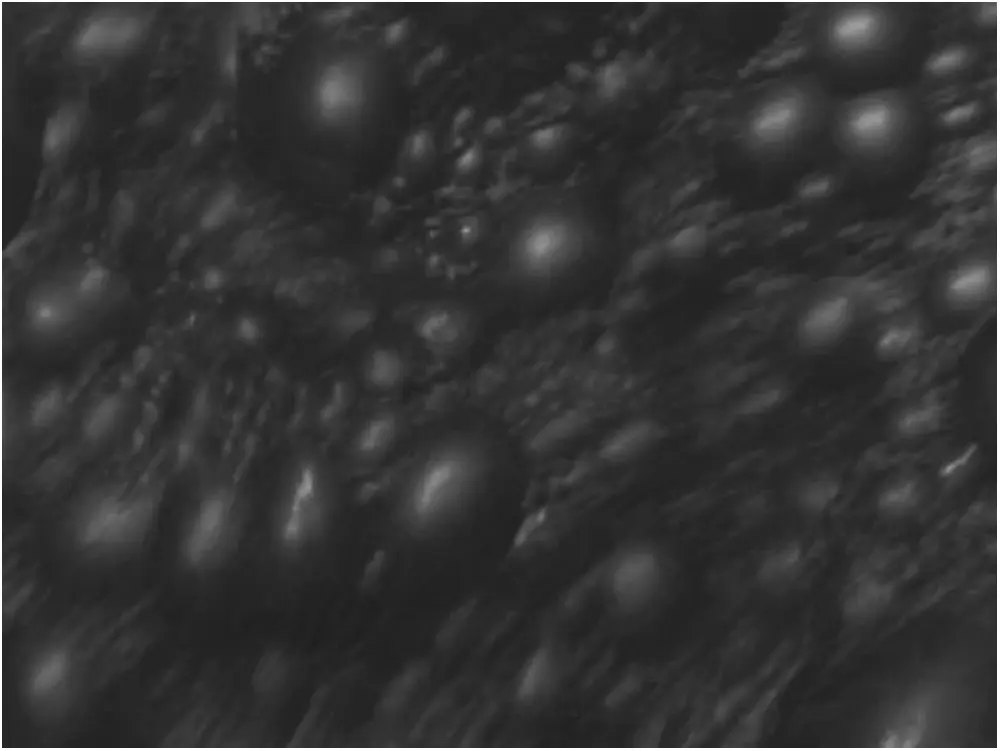Figure 1: Original imageFigure 2: Quality enhanced image(xx)

As can be seen from Figs. 3 and 4, the result of image processing using Hessian matrices in the yx and xy directions is that most of the linear structure in the image has been enhanced. As can be seen from Figs. 2 and 5, the result of image processing using Hessian matrices in the xx and yy directions is that most of the linear structure in the image is reduced. This result may be related to the size of the derivation window and the choice of Gaussian template variance.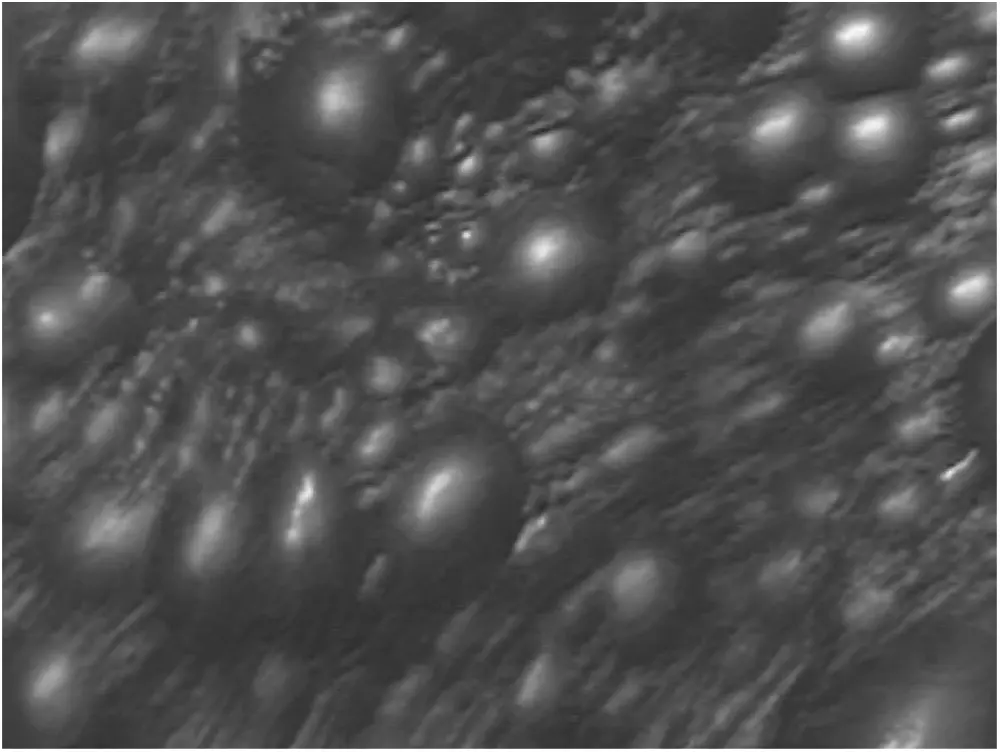Figure 3: Quality enhanced image(xy)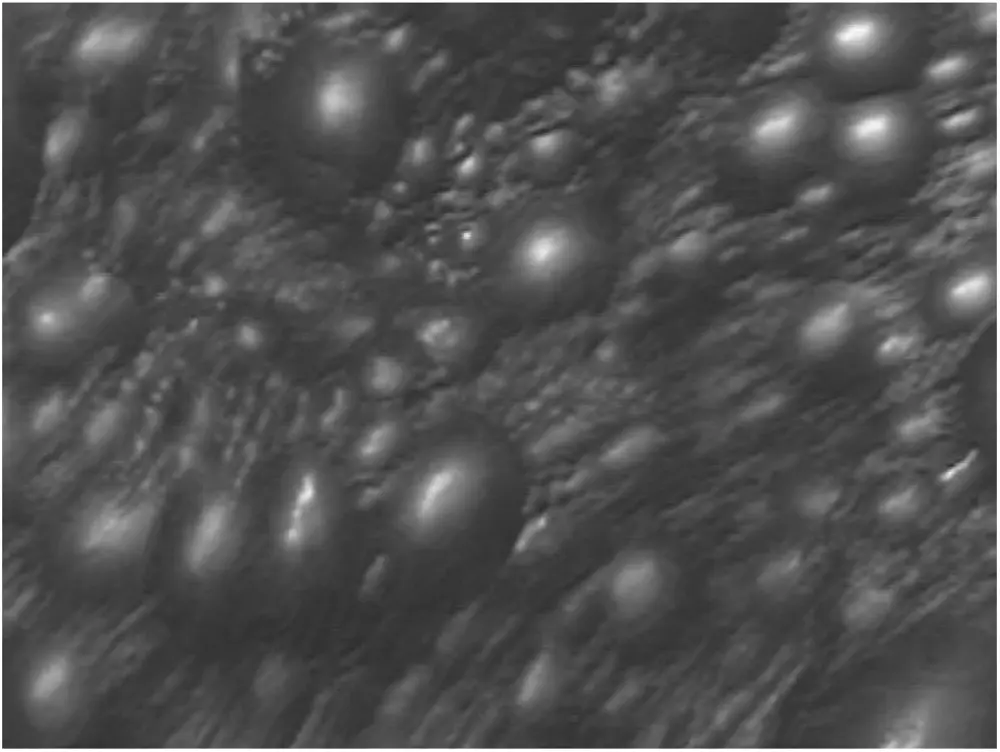Figure 4: Quality enhanced image(yx)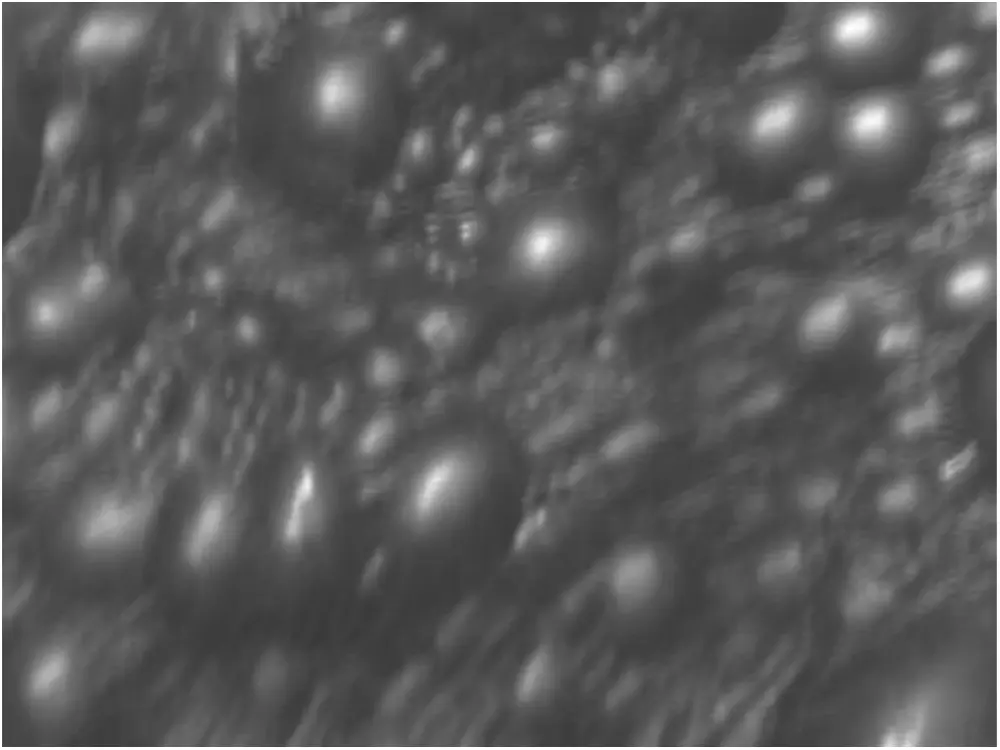Figure 5: Quality enhanced image(yy)

5  Conclusion

This paper proposes an image enhancement algorithm based on Hessian matrix. The simulation experiment results show that the linear structure of some images is enhanced, and the linear structure of some images is weakened. The effect of linear result enhancement is related to the size of the derivation window. However, in the same image, the thickness of the linear structure is different, and the same window size cannot be applied to all windows. The multi-template method may be able to solve the above problem, that is, convolve a point with Gaussian templates of multiple scales, and then select the most anisotropic result as the output of the point.

Acknowledgement: Thanks to Hunan First Normal University for providing experimental sites and equipment.

Funding Statement: This work was supported by the key scientific research projects of the Hunan Provincial Department of Education (No.19A099, 20A102), the Educational Reform Project of the Hunan Provincial Department of Education (No.HNJG-2021-1121), the Hunan First Normal University Teaching Reform Project (No.XYS21J09), Shaoyang City Science and Technology Bureau Science and Technology Research Project (No.2020GX31), Shaoyang University Cooperation Project (No.2019HX115).

Conflicts of Interest: The authors declare that they have no conflicts of interest to report regarding the present study.

## References

1. Chen, W. K., Zhang, Y. K., Jiang, B., Fang, W. H., & Cui, G. L. (2020). Efficient construction of excited-state hessian matrices with machine learning accelerated multilayer energy-based fragment method. The Journal of Physical Chemistry A, 124(27), 5684-5695. [Google Scholar]
2. Fu, S. C., Liu, W. F., Tao, D. P., Zhou, Y. C., & Nie, L. Q. (2020). HesGCN: Hessian graph convolutional networks for semi-supervised classification. Information Sciences, 514, 484-498. [Google Scholar]
3. Wang, X. Y., Ding, S. F., & Jia, W. K. (2020). Active constraint spectral clustering based on Hessian matrix. Soft Computing, 24(3), 2381-2390. [Google Scholar]
4. Hermes Eric, D. ., Khachik, S., Najm Habib, N. ., & Judit, Z. (2019). Accelerated saddle point refinement through full exploitation of partial Hessian diagonalization. Journal of Chemical Theory and Computation, 15(11), 6536-6549. [Google Scholar]
5. Azam, M. A., Khan, K. B., Ahmad, M., & Mazzara, M. (2021). Multimodal medical image registration and fusion for quality enhancement. Computers, Materials & Continua, 68(1), 821-840. [Google Scholar]
6. Chen, X., Chen, J., & Sha, Z. (2020). Edge detection based on generative adversarial networks. Journal of New Media, 2(2), 61-77. [Google Scholar]
7. Yang, T., Jia, S., Yang, B., & Kan, C. (2021). Research on tracking and registration algorithm based on natural feature point. Intelligent Automation & Soft Computing, 28(3), 683-692. [Google Scholar]
8. Sun, Z. Y., & Sugie, T. (2019). Identification of Hessian matrix in distributed gradient-based multi-agent coordination control systems. Numerical Algebra, Control & Optimization, 9(3), 297-318. [Google Scholar]
9. Petra, C. G., Qiang, F., & Lubin, M. (2018). On efficient Hessian computation using the edge pushing algorithm in Julia. Optimization Methods and Software, 33(4–6), 1010-1029. [Google Scholar]
10. Deng, L. Z., Zhu, H., Yang, Z., & Li, Y. J. (2019). Hessian matrix-based fourth-order anisotropic diffusion filter for image denoising. Optics and Laser Technology, 110, 184-190. [Google Scholar]
11. Hanbay, K., & Talu, M. F. (2018). A novel active contour model for medical images via the Hessian matrix and eigenvalues. Computers and Mathematics with Applications, 75(9), 3081-3104. [Google Scholar]
12. Wan, T., Shang, X. Q., Yang, W. L., Chen, J. H., & Li, D. Y. (2018). Automated coronary artery tree segmentation in X-ray angiography using improved Hessian based enhancement and statistical region merging. Computer Methods and Programs in Biomedicine, 157, 179-190. [Google Scholar]
13. Chahine, C., Vachier-Lagorre, C., & Chenoune, Y. (2018). Information fusion for unsupervised image segmentation using stochastic watershed and Hessian matrix. IET Image Processing, 12(4), 525-531. [Google Scholar]This work is licensed under a Creative Commons Attribution 4.0 International License, which permits unrestricted use, distribution, and reproduction in any medium, provided the original work is properly cited.